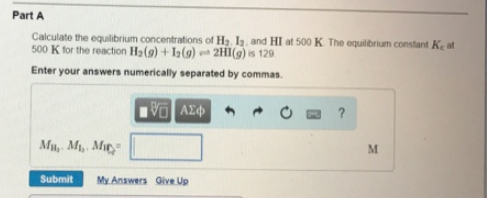# Problem: Calculate the equilibrium concentrations of H2, I2 and HI at 500K. The equilibrium constant Kc at 500K for the reaction H2(g) + I2(g) ⇌ 2HI(g) is 129.

###### FREE Expert Solution
94% (314 ratings)###### Problem Details

Calculate the equilibrium concentrations of H2, I2 and HI at 500K. The equilibrium constant Kc at 500K for the reaction H2(g) + I2(g) ⇌ 2HI(g) is 129.